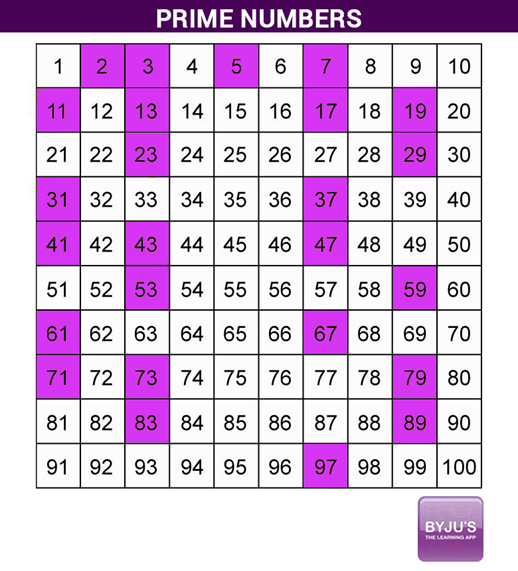# Is 61 a Prime Number

Is 61 a prime number? Before answering this question, let’s understand what a prime number is and how to identify whether a number is prime or not. There are numbers, having exactly two factors 1 and the number itself. Such numbers are 2, 3, 5, 7, 11, etc. These numbers are prime numbers. Mathematically, the numbers other than 1 whose only factors are 1 and the number itself are called Prime numbers. In contrast, numbers having more than two factors are called Composite numbers. For example, the numbers 4, 6, 8, 10, 12, etc are composite. However, 1 is neither a prime nor a composite number.

 Also, check:

## Is 61 Prime or Composite?

In the above section, you have learned what is a prime and composite number. Now the question is whether the number 61 is prime or not. Yes, 61 is a prime number. By the definition of prime numbers, if a number has only 2 factors, i.e. 1 and the number itself, then it is a prime number. If the number has more than 2 factors, then it is called a composite number.

### How is 61 a Prime Number?

To understand how 61 becomes a prime number, one should find the factors of 61. As we know, the factors of any number can be found either by the prime factorisation method or division method. In both of these cases, we get a pair of numbers which get multiplied and produce the result as the original number. Thus, the pairs of numbers, which produce 61 on multiplication are given as:

1 × 61 = 61

Or

61 × 1 = 61

Here, the number 61 has only two unique numbers that produce the result as 61 on multiplication. Also, these two numbers are 1 and the number itself, i.e. 61.

Therefore, 61 is called a prime number.

Also, get the related questions on classification of the number 61 in the table given below:

 Is 61 a prime number? Yes Is 61 a composite number? No What are the factors for 61? 1 and 61 What is (are) prime factors of 61? 61 only Is 61 a perfect square? No Is 61 a perfect cube number? No Is 61 an odd or even number? Odd number What can divide 61 evenly? The factors of 61 divide it evenly, i.e. 1 and 61

Let’s have a look at the prime numbers chart below to know which numbers before and after 61 are prime numbers that are below 100.From the above, one can answer the question, Is 61 a Prime number or Not? However, the answer for the question is 61 is a prime number.

Apart from the above specified ways, there are two more methods to check whether 61 is a prime number or not.

Method 1:

6(10) + 1 = 60 + 1 = 61

That means, 61 can be expressed in the form 6n + 1, where n is a natural number. Hence, it is a prime number.

Method 2:

To know the prime numbers greater than 40, we can use the formula below:

n2 + n + 41, where n = 0, 1, 2, ….., 39

In the above formula, by substituting n = 4, we get;

(4)2 + 4 + 41 = 16 + 4 + 41 = 61

Thus, we can say that 61 is a prime number.

## Frequently Asked Questions – FAQs

### What are the factors of 61?

The factors of 61 are 1 and 61.

### Is 61 a prime number yes or no?

Yes, 61 is a prime number.

### Is 61 a prime number? Explain why.

Yes, 61 is a prime number since it has only two factors namely one and the number itself, i.e. 1 and 61. So as per the definition of prime numbers, 61 is considered as a prime number.

### Is 61 a prime number or composite number?

Yes, 61 is a prime number but not a composite number. We know that composite numbers must contain more than two factors, whereas 61 has exactly two factors.

### What is the fastest way to find a prime number?

To find whether a number is prime or composite, we need to get the factors of it. Based on the number of factors, we can tell whether the number is a prime number or not. Also, we have some specified formulas in maths to find the list of prime numbers.